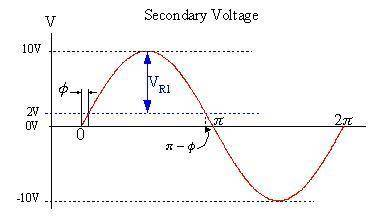# Help with resistor power dissipation homework

This question is in reference to the diagram here:
http://imageshack.us/f/17/ecediagram.jpg/

Find the average power dissipated on the resistor.
Assume Vth for the diodes is 1V, N1 = 1000, N2 = 100, the transformer is ideal (non-lossy), and V(t) is a purely sinusoidal voltage with frequency of 50 Hz and amplitude of 100V.

I've tried working it out several times, but I can't figure out exactly what to integrate. Thanks for your help!

gneill
Mentor
Hi thepcphysicia, welcome to PF.

You'll need to show some attempt at the problem before we can give you any help. Have you determined the waveform of the voltage (or current) that will appear across R1?

Hi,

Here is my work thus far:
http://imageshack.us/photo/my-images/202/photo2la.jpg/

The waveform of the voltage is given by 10*sin(100pi*t). I think that it is squared, because P=V^2/R.

The waveform is also shifted down two due to the voltage drop across the diodes.

Anybody? The assignment is due in a few hours...

gneill
Mentor
You might want to consider the period of the sinewave in terms of angle rather than time, and do the integration symbolically rather than carrying all those decimals around and fiddling with the milliseconds and their fractions. Then the period of a cycle is just $1/(2\pi)$, and you can call the "turn-on angle" for the the diodes $\phi$, given by:
$$10V sin(\phi) = 2V$$The voltage that appears across the resistor R1 is shown in the diagram. In your proposed solution you made it $(10 sin(stuff))^2 - 2)$, but that's not quite right; The 2V needs to be taken from the sin() before squaring occurs.

#### Attachments

Last edited:
Awesome, thank you SOOO much! The diagram was incredibly helpful!